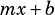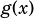Is there a way to solve this system of equations without using the quadratic formula (or graphing)?

f(x) = –2/3 x + 4
g(x) = 3(x + 2)^2 – 4

How many solutions does the system above have?

It seems like this question doesn’t actually require you to solve the system, just figure out how many solutions it has! So yes, as long as you know a bit about standard equations, you’re set without graphing or really much math at all.

The equation foris in slope-intercept () form, so we know that it’s a line with a slope ofand a-intercept of 4.

The equation foris for a parabola in vertex form, so we know it’s a parabola that opens upwards and has its vertex at.

If you think about these things together you should see the answer. A line with a negative slope and a positive-intercept will have two intersections with a parabola that 1) has its vertex below the line and 2) opens upwards. And two intersections means two solutions.